# How to Make an Age Calculator in Python

Learn how you to build an age calculator that calculates your age based on your birthday using Tkinter and datetime library in Python.
12 min read · Updated mar 2023 · GUI Programming

Before we get started, have you tried our new Python Code Assistant? It's like having an expert coder at your fingertips. Check it out!

Before we dive deeper into this article, let us first understand what an age calculator app is; this app calculates the user's age using their birth date (day, month, and year).

This article has been split into two sections. In the first section, we will build a command line version, and finally, in the second section, we will make a GUI version of the app. We will build all these from the ground up.

## Setting up the Environment

First things first, let us set up the environment, create two new Python files and call them `age_calculator_cli.py` and `age_calculator_ui.py`: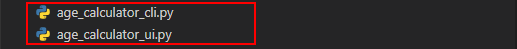You can call them anything per your preference; make sure the file names are meaningful.

## Building the Command Line Version

Now that the environment is all set, open the `age_calculator_cli.py` file, and do the following import:

``from datetime import date``

Here we are importing `date` from `datetime`, this module provides several functions and classes for handling dates and times.

We will now create a function for calculating the age; we will call it `calculate_age()`. Just below the import, paste these lines of code:

``````# defining the for calculating the age, the function takes day
def calculate_age(day, month, year):
# we are getting the current date using the today()
today = date.today()
# convering year, month and day into birthdate
birthdate = date(year, month, day)
# calculating the age
age = today.year - birthdate.year - ((today.month, today.day) < (birthdate.month, birthdate.day))
# return the age value
return age``````

In the above function, we are passing three arguments to the function, `date`, `month`, and `year`, we will get these arguments from the user.

Inside the function, we are getting the current date from `datetime` module using the `today()` function. After that, we create a `birthdate` variable using the `date()` function; it takes a `year`, `month`, and `day` as arguments. From there, we are now calculating the `age` by doing some subtractions, the code:

``((today.month, today.day) < (birthdate.month, birthdate.day))``

Checks if the day or month of the variable `today` precedes the day or month of the `birthdate` variable, the return value is a boolean value, and finally, we return the calculated `age`.

Now we need to get the `day`, `month`, and `year` from the user, just after the function definition, paste these lines of code:

``````# the try/except block
# the try will execute if there are no exceptions
try:
# we are getting day, month, and year using input() function
day = input('Enter day:')
month = input('Enter month:')
year = input('Enter year:')
# creating a variable called age_result and we are also calling the claculate_age function
age_result = calculate_age(int(day), int(month), int(year))
print(f'You are {age_result} years old')

# the except will catch all errors
except:
print(f'Failed to calculate age, either day or month or year is invalid')``````

Here we have a `try/except` block, inside the `try` statement, we have code for getting data from the user; this is done using the `input()` function, and with that user data, we calculate the age via the function call. If the code execution encounters any errors, the `except` statement will come to the rescue.

To test the program, use the following:

``\$ python age_calculator_cli.py``

The output is as follows if you enter valid data:

``````Enter day:12
Enter month:10
Enter year:2000
You are 21 years old``````

The program is running as expected, rerun it, but this time, let's enter invalid data. The output will be as below:

``````Enter day:-1
Enter month:h
Enter year:0.5
Failed to calculate age, either day or month or year is invalid``````

We have successfully created the command line version of the age calculator!

## Building the GUI Version

In this section, we will now focus on building the Tkinter version of our age calculator. Its functionality is not different from the command line version. The only difference is that the Tkinter version app has a user interface that allows users to enter data. This is what we are going to build at the end of this section: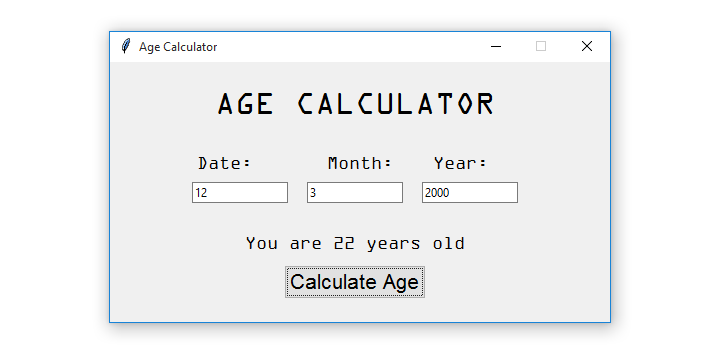So without further ado, let us start building the app.

## Designing the User Interface

Before everything else, let us start by designing the user interface, do the following necessary imports as follows in the `age_calculator_ui.py`:

``````from tkinter import *
from tkinter import ttk
from tkinter.messagebox import showerror
from datetime import date``````

Here is what the imports mean; starting with the first one, we are importing everything from `tkinter`. To do this, we use an asterisk or a wildcard. After that, we import `ttk` from `tkinter`, `ttk` is for styling widgets (labels, buttons, entries, etc.).

We are also importing the `showerror` message box for displaying error messages to the user from `tkinter.messagebox`. Finally, we are importing the `datetime` module.

We will create the window just after the imports. Paste these lines of code:

``````# creating the main window
window = Tk()
# the title for the window
window.title('Age Calculator')
# the dimensions and position of the windodw
window.geometry('500x260+430+300')
# making the window nonresizabale
window.resizable(height=FALSE, width=FALSE)

# runs the window infinitely until uses closes it
window.mainloop()``````

For the sake of being on the same page, let us break the code a bit. Firstly, we are creating the main window using the `Tk()` function that comes with Tkinter, then we give the window the title using the `title(`) function, we are then defining the dimensions (500x260) and the position (430+300) for the window using the `geometry()` function, to make the window non-resizable, we are using the `resizable()` function with `height` and `width` set to `FALSE`.

Running the program, you will get the following: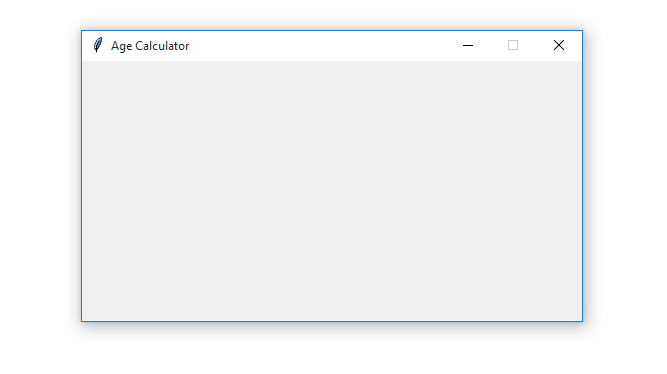After creating the window, we now need to make the container for all the widgets; we will use a `Canvas` widget. This `Canvas` will be inside the main window, and it will take up the `height` and `width` like this: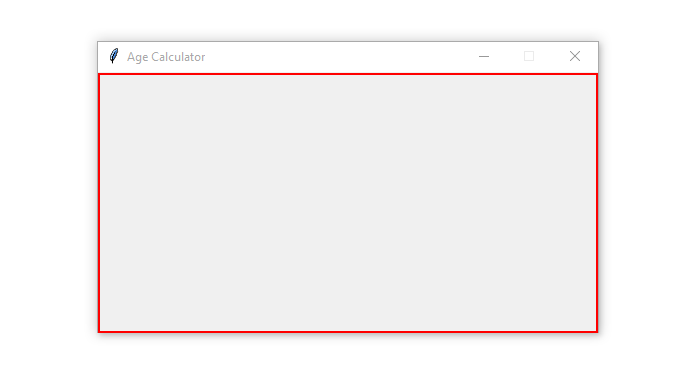So just after:

``window.resizable(height=FALSE, width=FALSE)``

Paste this code:

``````# the canvas to contain all the widgets
canvas = Canvas(window, width=500, height=400)
canvas.pack()``````

We are creating a canvas using the `Canvas()` function, and placing it inside the main window. For the size of the canvas, we are using `width` and `height`.

Now we need to create widgets that will be placed inside the created canvas, but before making the widgets, let us create their styles; just below the canvas definition, add these lines of code:

``````# ttk styles for the labels
label_style = ttk.Style()
label_style.configure('TLabel', foreground='#000000', font=('OCR A Extended', 14))

# ttk styles for the button
button_style = ttk.Style()
button_style.configure('TButton', foreground='#000000', font=('DotumChe', 16))

# ttk styles for the entries
entry_style = ttk.Style()
entry_style.configure('TEntry', font=('Dotum', 15))``````

In the code above, we are creating styles for the widgets; we are using the `ttk` module for this.

We are building a style object using `ttk.Style()` function, so to add styles to the object, we will use the `configure()` function, this function takes the style name (`TLabel`, `TButton`, or `TEntry`), `foreground`, and `font()` as some of its arguments.

In our widgets, we will use the style names to refer to or point to the styles we want. Now that the styles are taken care of, we will create the widgets. Let’s start with the label for displaying the large text. Below the styles, add this code:

``````# the label for displaying the big text
big_label = Label(window, text='AGE CALCULATOR', font=('OCR A Extended', 25))

# placing the big label inside the canvas
canvas.create_window(245, 40, window=big_label)``````

The code creates a label using `Label()`, we are passing `window`, `text`, and `font` as arguments, something to note here, this label has independent styles; it is not using the styles we have created.

Below the label, we are adding it to the canvas using the `create_window()` function, this function takes two integers (245 and 40) and a `window` as arguments, the first integer positions the label horizontally, and the second integer positions it vertically.

Running the program, you will get this: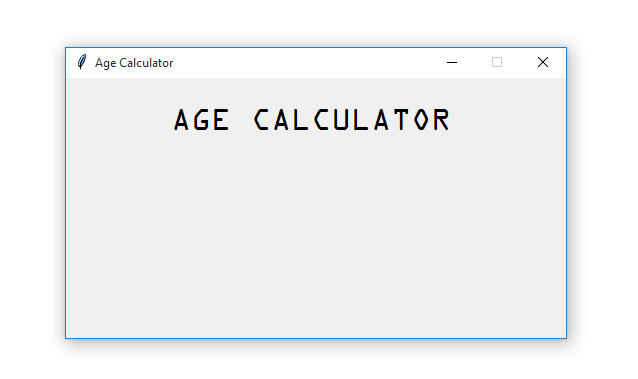So far, so good. Our app is taking shape; now let us create the remaining widgets; add this code below the `big_label`:

``````# label and entry for the day
day_label = ttk.Label(window, text='Day:', style='TLabel')
day_entry = ttk.Entry(window, width=15, style='TEntry')
# label and entry for the month
month_label = ttk.Label(window, text='Month:', style='TLabel')
month_entry = ttk.Entry(window, width=15, style='TEntry')
# label and entry for the year
year_label = ttk.Label(window, text='Year:', style='TLabel')
year_entry = ttk.Entry(window, width=15, style='TEntry')
# the button
calculate_button = ttk.Button(window, text='Calculate Age', style='TButton', command=calculate_age)
# label for display the calculated age
age_result = ttk.Label(window, text='', style='TLabel')
# adding the day label and entry inside the canvas
canvas.create_window(114, 100, window=day_label)
canvas.create_window(130, 130, window=day_entry)
# adding the month label and entry inside the canvas
canvas.create_window(250, 100, window=month_label)
canvas.create_window(245, 130, window=month_entry)
# adding the year label and entry inside the canvas
canvas.create_window(350, 100, window=year_label)
canvas.create_window(360, 130, window=year_entry)
# adding the age_result and entry inside the canvas
canvas.create_window(245, 180, window=age_result)
# adding the calculate button inside the canvas
canvas.create_window(245, 220, window=calculate_button)``````

With this code snippet, we are creating the following widgets:

• Labels for displaying `Day`, `Month`, `Year`, and `age_result` text:
• `day_label`
• `month_label`
• `year_label`
• `age_result`, this is empty for now.
• Entries for capturing `day`, `month` and `year` values:
• `day_entry`
• `month_entry`
• `year_entry`
• A button:
• `calculate_button`

All these widgets are `ttk` widgets, so to style them, we are passing the `style` argument, for example, the button widget uses the `TButton` style that we created.

Testing the app, we get the following: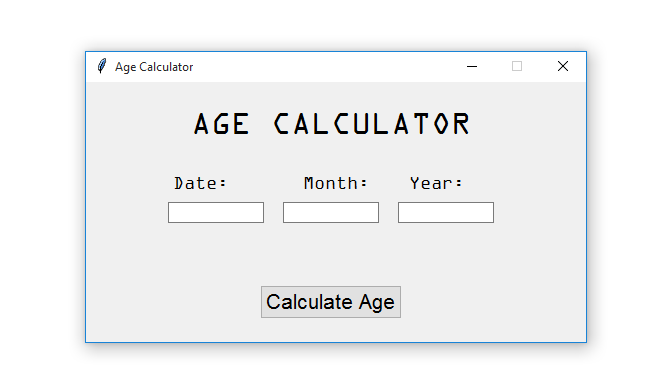Congratulations on successfully designing the app’s user interface!

## Implementing the Age Calculation Functionality

Now that the user interface is taken care of, let us make the app reactive. The app should be able to get data from the user and calculate the age. To do this, we will create a function just below the imports called `calculate_age()`, paste this code:

``````# the function for calculating the age
def calculate_age():
# the try/except block
try:
# getting current date
today = date.today()
# getting day from the day entry
day = int(day_entry.get())
# # getting month from the month entry
month = int(month_entry.get())
# getting year from the year entry
year = int(year_entry.get())
# creating a date object
birthdate = date(year, month, day)
# calculating the age
age = today.year - birthdate.year - ((today.month, today.day) < (birthdate.month, birthdate.day))
# displaying the age using the age result label
age_result.config(text='You are ' + str(age) + ' years old')
# if an error occurs the showerror window will pop up
except:
showerror(title='Error', message='An error occurred while trying to ' \
'calculate age\nThe following could ' \
'be the causes:\n->Invalid input data\n->An empty field/fields\n'\
'Make sure you enter valid data and fill all the fields')``````

This function is all the same as the one we used in the command line version of the app; the only difference here is that in the `try` statement, we are getting the `day`, `month`, and `year` from the entries using the `get()` function and also in the `except` statement, all errors that the app will catch will be displayed on the `showerror` popup window.

Nothing happens if you run the app, enter valid data in the entries, and click the button. The reason is that we have not linked the `calculate_age()` function with the button. We want the function to be triggered once the user clicks the button. To do this, we will use the command argument that comes with the button widget, edit the `calculate_age` button code and make it look like this:

``calculate_button = ttk.Button(window, text='Calculate Age', style='TButton', command=calculate_age)``

Rerun the app, re-enter the valid data, and you will get the following: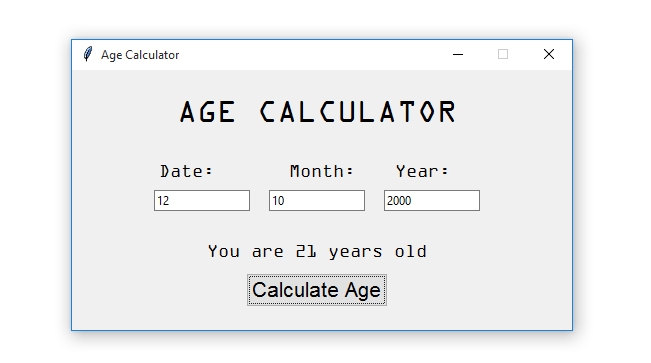What if the user enters invalid data or leaves one or all the entries empty and clicks the button? The output will be this: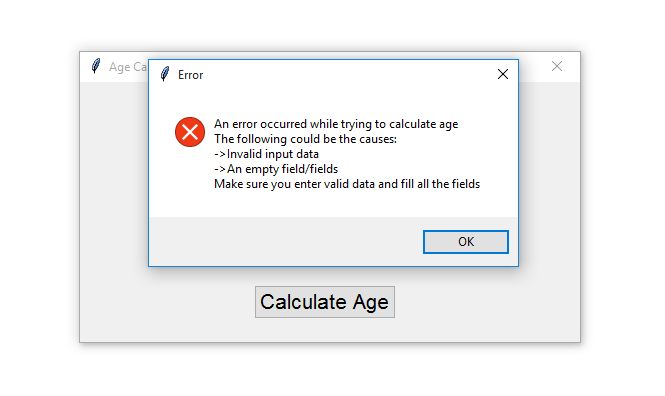This means that the app is working perfectly!

## Conclusion

This article showed you how to create an age calculator using Python. We walked you through building two versions of the age calculator app, the command line and the Tkinter version. We hope you will use the knowledge you acquired from this article in your future projects!

You can get the complete code here.

Here are some related apps we've built in other tutorials:

Happy coding ♥

Loved the article? You'll love our Code Converter even more! It's your secret weapon for effortless coding. Give it a whirl!

Sharing is caring!

### Comment panel

Got a coding query or need some guidance before you comment? Check out our Python Code Assistant for expert advice and handy tips. It's like having a coding tutor right in your fingertips!